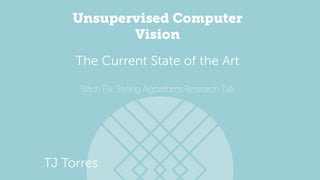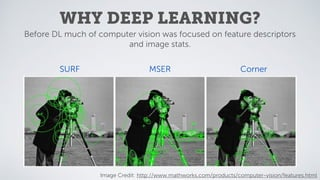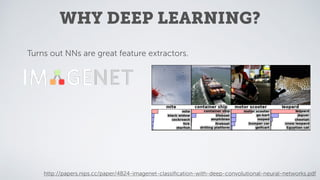Successfully reported this slideshow.

# Unsupervised Computer Vision: The Current State of the Art×

# Unsupervised Computer Vision: The Current State of the Art

This presentation was originally given at a styling research presentation at Stitch Fix, where I talk about some of the recent progress in the field of unsupervised deep learning methods for image analysis. It includes descriptions of Variational Autoencoders (VAE), Generative Adversarial Networks (GAN), their hybrid (VAE/GAN), Generative Moment Matching Networks (GMMN), and Adversarial Autoencoders.

This presentation was originally given at a styling research presentation at Stitch Fix, where I talk about some of the recent progress in the field of unsupervised deep learning methods for image analysis. It includes descriptions of Variational Autoencoders (VAE), Generative Adversarial Networks (GAN), their hybrid (VAE/GAN), Generative Moment Matching Networks (GMMN), and Adversarial Autoencoders.

### Unsupervised Computer Vision: The Current State of the Art

1. 1. Unsupervised Computer Vision Stitch Fix, Styling Algorithms Research Talk The Current State of the Art TJ Torres Data Scientist, Stitch Fix
2. 2. WHY DEEP LEARNING? Before DL much of computer vision was focused on feature descriptors and image stats. SURF MSER Corner Image Credit: http://www.mathworks.com/products/computer-vision/features.html
3. 3. WHY DEEP LEARNING? Turns out NNs are great feature extractors. http://papers.nips.cc/paper/4824-imagenet-classiﬁcation-with-deep-convolutional-neural-networks.pdf
4. 4. WHY DEEP LEARNING? Turns out NNs are great feature extractors. Team name Entry description Classiﬁcation error Localization error GoogLeNet No localization. Top5 val score is 6.66% error. 0.06656 0.606257 VGG a combination of multiple ConvNets, including a net trained on images of different size (fusion weights learnt on the validation set); detected boxes were not updated 0.07325 0.256167 VGG a combination of multiple ConvNets, including a net trained on images of different size (fusion done by averaging); detected boxes were not updated 0.07337 0.255431 VGG a combination of multiple ConvNets (by averaging) 0.07405 0.253231 VGG a combination of multiple ConvNets (fusion weights learnt on the validation set) 0.07407 0.253501 Leaderboard
5. 5. WHY DEEP LEARNING? Turns out NNs are great feature extractors. Team name Entry description Classiﬁcation error Localization error GoogLeNet No localization. Top5 val score is 6.66% error. 0.06656 0.606257 VGG a combination of multiple ConvNets, including a net trained on images of different size (fusion weights learnt on the validation set); detected boxes were not updated 0.07325 0.256167 VGG a combination of multiple ConvNets, including a net trained on images of different size (fusion done by averaging); detected boxes were not updated 0.07337 0.255431 VGG a combination of multiple ConvNets (by averaging) 0.07405 0.253231 VGG a combination of multiple ConvNets (fusion weights learnt on the validation set) 0.07407 0.253501 Leaderboard Convolution: gives local, translation invariant feature hierarchy
6. 6. WHY DEEP LEARNING? Image Credit: http://deeplearning.stanford.edu/wiki/index.php/Feature_extraction_using_convolution
7. 7. WHY DEEP LEARNING? Edges Curves Top of 3 shapes Softmax Output: Classiﬁcation Image Credit: http://parse.ele.tue.nl/education/cluster2
8. 8. WHY DEEP LEARNING? Image Credit: http://blog.keras.io/how-convolutional-neural-networks-see-the-world.html
9. 9. WHY DEEP LEARNING? Image Credit: http://blog.keras.io/how-convolutional-neural-networks-see-the-world.html
10. 10. WHY DEEP LEARNING? Image Credit: http://blog.keras.io/how-convolutional-neural-networks-see-the-world.html
11. 11. WHY DEEP LEARNING? Image Credit: http://blog.keras.io/how-convolutional-neural-networks-see-the-world.html
12. 12. WHY DEEP LEARNING? Image Credit: http://blog.keras.io/how-convolutional-neural-networks-see-the-world.html
14. 14. WHY UNSUPERVISED? Unfortunately very few image sets come with labels.
15. 15. WHY UNSUPERVISED? Unfortunately very few image sets come with labels. What are the best labels for fashion/style?
16. 16. THE UNSUPERVISED MO Try to learn embedding space of image data. (generally includes generative process)
17. 17. THE UNSUPERVISED MO Try to learn embedding space of image data. (generally includes generative process) 1) Train encoder and decoder to encode then reconstruct image.
18. 18. THE UNSUPERVISED MO Try to learn embedding space of image data. (generally includes generative process) 1) Train encoder and decoder to encode then reconstruct image. 2) Generate image from random embedding and reinforce “good” looking images.
19. 19. THE UNSUPERVISED MO Try to learn embedding space of image data. (generally includes generative process) 1) Train encoder and decoder to encode then reconstruct image. 2) Generate image from random embedding and reinforce “good” looking images. DOWNSIDES Higher dimension embeddings = Non-interpretable Latent distributions may contain gaps. No sensible continuum.
20. 20. OUTLINE 1. Variational Auto-encoders (VAE) 2. Generative Adversarial Networks (GAN) 3. The combination of the two (VAE/GAN) 4. Generative Moment Matching Networks (GMMN) 5. Adversarial Auto-encoders (AAE?) Brieﬂy
21. 21. OUTLINE 1. Variational Auto-encoders (VAE) 2. Generative Adversarial Networks (GAN) 3. The combination of the two (VAE/GAN) 4. Generative Moment Matching Networks (GMMN) 5. Adversarial Auto-encoders (AAE?) Brieﬂy stitchﬁx/fauxtograph
22. 22. VARIATIONAL AUTO-ENCODERS
23. 23. ENCODING input Convolution
24. 24. input ENCODING Convolution
25. 25. latent ENCODING Convolution
26. 26. VARIATIONAL STEP sample from distribution }µ } q (z) = N(z; µ(i) , 2(i) I)
27. 27. VARIATIONAL STEP sampled Deconvolution
28. 28. DECODING output Deconvolution
29. 29. DECODING reconstruction Deconvolution
30. 30. CALCULATE LOSS L(x) = DKL(q (z)||N(0, I)) + MSE(x, yout)
31. 31. UPDATE WEIGHTS W (l)⇤ ij = W (l) ij ✓ 1 ↵ @L @Wij ◆ @L @W (l) ij = ✓ @L @xout ◆ ✓ @xout @f(n 1) ◆ · · · @f(l) @W (l) ij !
32. 32. source: @genekogan Because of pixel-wise MSE loss. Non-centered features disproportionately penalized. OUTPUT
33. 33. source: @genekogan Because of pixel-wise MSE loss. Non-centered features disproportionately penalized. OUTPUT Note Blurring hair.
35. 35. GAN STRUCTURE Latent Random Vector Generator Discriminator
36. 36. Discriminator GAN STRUCTURE Generator Filtered
37. 37. Discriminator GAN STRUCTURE Generator Image
38. 38. Discriminator GAN STRUCTURE Generator Gen/Train Image
39. 39. Discriminator GAN STRUCTURE Generator Filtered
40. 40. Discriminator GAN STRUCTURE Generator Yes/No
41. 41. Discriminator TRAINING Generator Generator and Discriminator play minimax game. min G max D V (D, G) = Ex⇠pdata(x) [log D(x)] + Ez⇠pz(z) [log(1 D(G(z)))]
42. 42. Discriminator TRAINING Generator Lower loss for fooling Discriminator. Generator and Discriminator play minimax game. Lower loss for IDing correct training/generated data. min G max D V (D, G) = Ex⇠pdata(x) [log D(x)] + Ez⇠pz(z) [log(1 D(G(z)))]
43. 43. Discriminator TRAINING Generator Lower loss for fooling Discriminator. Generator and Discriminator play minimax game. Lower loss for IDing correct training/generated data. LD = 1 m mX i=1 h log D ⇣ x(i) ⌘ + log ⇣ 1 D ⇣ G ⇣ z(i) ⌘⌘⌘i LG = 1 m mX i=1 log ⇣ 1 D ⇣ G ⇣ z(i) ⌘⌘⌘ min G max D V (D, G) = Ex⇠pdata(x) [log D(x)] + Ez⇠pz(z) [log(1 D(G(z)))]
44. 44. Discriminator TRAINING Generator Lower loss for fooling Discriminator. Generator and Discriminator play minimax game. Lower loss for IDing correct training/generated data. LD = 1 m mX i=1 h log D ⇣ x(i) ⌘ + log ⇣ 1 D ⇣ G ⇣ z(i) ⌘⌘⌘i LG = 1 m mX i=1 log ⇣ 1 D ⇣ G ⇣ z(i) ⌘⌘⌘ http://arxiv.org/pdf/1406.2661v1.pdf
45. 45. OUTPUT http://arxiv.org/pdf/1511.06434v2.pdf
46. 46. OUTPUT http://arxiv.org/pdf/1511.06434v2.pdf
47. 47. OUTPUT http://arxiv.org/pdf/1511.06434v2.pdf
48. 48. OUTPUT http://arxiv.org/pdf/1511.06434v2.pdf Unfortunately Only Generative
49. 49. VAE+GAN
50. 50. VAE+GAN STRUCTURE Generator DiscriminatorEncoder O
51. 51. VAE+GAN STRUCTURE Generator DiscriminatorEncoder O S
52. 52. VAE+GAN STRUCTURE Generator DiscriminatorEncoder O S O G(S) G(E(O))
53. 53. VAE+GAN STRUCTURE Generator DiscriminatorEncoder O S O G(S) G(E(O))
54. 54. VAE+GAN STRUCTURE Generator DiscriminatorEncoder O S O G(S) G(E(O)) Yes/ No MSE
55. 55. Discriminator TRAINING Generator Train Encoder, Generator and Discriminator with separate optimizers. Encoder LE = DKL(q (z)||N(0, I)) + MSE(Dl(x), Dl(G(E(x)))) LG = ⇥ MSE(Dl(x), Dl(G(E(x)))) LGAN LD = LGAN = || log(D(x)) + log(1 D(E(G(x)))) + log(1 D(G(z)))||1
56. 56. Discriminator TRAINING Generator Train Encoder, Generator and Discriminator with separate optimizers. Encoder LE = DKL(q (z)||N(0, I)) + MSE(Dl(x), Dl(G(E(x)))) LG = ⇥ MSE(Dl(x), Dl(G(E(x)))) LGAN LD = LGAN = || log(D(x)) + log(1 D(E(G(x)))) + log(1 D(G(z)))||1 VAE Prior learned similarity learned similarity GAN GAN discriminator loss
57. 57. OUTPUT http://arxiv.org/pdf/1512.09300v1.pdf
58. 58. OUTPUT http://arxiv.org/pdf/1512.09300v1.pdf
59. 59. OUTPUT http://arxiv.org/pdf/1512.09300v1.pdf
60. 60. TAKEAWAY http://arxiv.org/pdf/1512.09300v1.pdf We are trying to get away from pixels to begin with so why use pixel distance as metric?
61. 61. TAKEAWAY http://arxiv.org/pdf/1512.09300v1.pdf Learned similarity metric provides feature-level distance rather than pixel-level. We are trying to get away from pixels to begin with so why use pixel distance as metric?
62. 62. TAKEAWAY http://arxiv.org/pdf/1512.09300v1.pdf Learned similarity metric provides feature-level distance rather than pixel-level. We are trying to get away from pixels to begin with so why use pixel distance as metric? Latent space of a GAN with the encoder of a VAE
63. 63. TAKEAWAY http://arxiv.org/pdf/1512.09300v1.pdf Learned similarity metric provides feature-level distance rather than pixel-level. We are trying to get away from pixels to begin with so why use pixel distance as metric? Latent space of a GAN with the encoder of a VAE …BUT NOT THAT EASY TO TRAIN
64. 64. GENERATIVE MOMENT MATCHING NETWORKS
65. 65. DESCRIPTION Use Maximum Mean Discrepancy between generated data and test data for loss. Train generative network to output distribution with moments matching dataset. LMMD2 = 1 N NX i=0 (xi) 1 M MX j=0 (yj) 2 LMMD2 = 1 N2 NX i=0 NX i0=0 k(xi, xi0 ) 2 MN NX i=0 MX j=0 k(xi, yj) + 1 M2 MX j=0 MX j0=0 k(yj, yj0 )
66. 66. DESCRIPTION
67. 67. OUTPUT http://arxiv.org/pdf/1502.02761v1.pdf